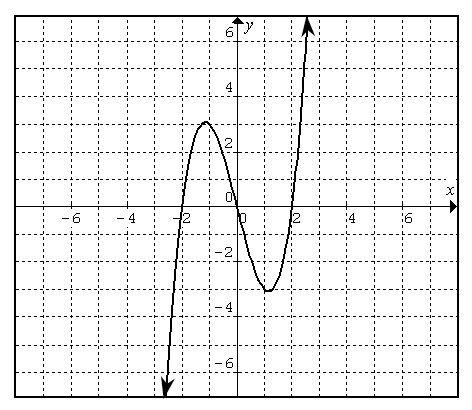### Home > PC > Chapter 6 > Lesson 6.1.2 > Problem6-34

6-34.

Given the graph of f(x) to the right, sketch the graph of: 6-34 HW eTool (Desmos)

1. $−f\left(x\right) + 2$

It is reflected vertically and shifted up 2 units.

2. $2f\left(−x\right)$

It is reflected horizontally and stretched vertically.

3. $\frac { 1 } { f ( x ) }$

Asymptotes are at $x = −2, 0,$ and $2$.Use the eTool below to visualize this problem.
Click on the link to the right to view the full version of the eTool: 6-34 HW eTool